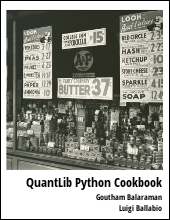This post will walk through an example of modeling fixed rate bonds using QuantLib Python.

Visit here for other QuantLib Python examples. If you found these posts useful, please take a minute by providing some feedback.

Let's consider a hypothetical bond with a par value of 100, that pays 6% coupon semi-annually issued on January 15th, 2015 and set to mature on January 15th, 2016. The bond will pay a coupon on July 15th, 2015 and January 15th, 2016. The par amount of 100 will also be paid on the January 15th, 2016.

To make things simpler, lets assume that we know the spot rates of the treasury as of January 15th, 2015. The annualized spot rates are 0.5% for 6 months and 0.7% for 1 year point. Lets calculate the fair value of this bond.

>>> 3/pow(1+0.005, 0.5) + (100 + 3)/(1+0.007)
105.27653992490681


Lets calculate the same thing using QuantLib.

>>> import QuantLib as ql
>>> todaysDate = ql.Date(15, 1, 2015)
>>> ql.Settings.instance().evaluationDate = todaysDate
>>> spotDates = [ql.Date(15, 1, 2015), ql.Date(15, 7, 2015), ql.Date(15, 1, 2016)]
>>> spotRates = [0.0, 0.005, 0.007]
>>> dayCount = ql.Thirty360()
>>> calendar = ql.UnitedStates()
>>> interpolation = ql.Linear()
>>> compounding = ql.Compounded
>>> compoundingFrequency = ql.Annual
>>> spotCurve = ql.ZeroCurve(spotDates, spotRates, dayCount, calendar, interpolation,
compounding, compoundingFrequency)
>>> spotCurveHandle = ql.YieldTermStructureHandle(spotCurve)


So far we have created the term structure and the variables are rather self explanatory. Now lets construct the fixed rate bond.

>>> issueDate = ql.Date(15, 1, 2015)
>>> maturityDate = ql.Date(15, 1, 2016)
>>> tenor = ql.Period(ql.Semiannual)
>>> calendar = ql.UnitedStates()
>>> dateGeneration = ql.DateGeneration.Backward
>>> monthEnd = False
>>> schedule = ql.Schedule (issueDate, maturityDate, tenor, calendar, bussinessConvention,
bussinessConvention , dateGeneration, monthEnd)
>>> list(schedule)
[Date(15, 1, 12015), Date(15,7,2015), Date(15,1,2016)]

# Now lets build the coupon
>>> dayCount = ql.Thirty360()
>>> couponRate = .06
>>> coupons = [couponRate]

# Now lets construct the FixedRateBond
>>> settlementDays = 0
>>> faceValue = 100
>>> fixedRateBond = ql.FixedRateBond(settlementDays, faceValue, schedule, coupons, dayCount)

# create a bond engine with the term structure as input;
# set the bond to use this bond engine
>>> bondEngine = ql.DiscountingBondEngine(spotCurveHandle)
>>> fixedRateBond.setPricingEngine(bondEngine)

# Finally the price
>>> fixedRateBond.NPV()
105.27653992490683


Voila!